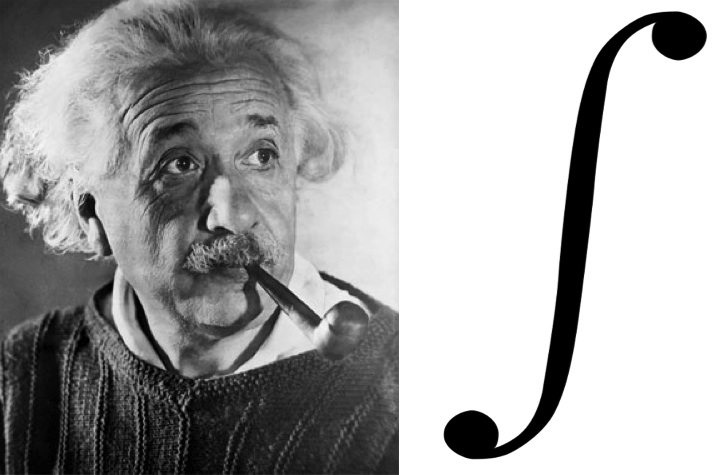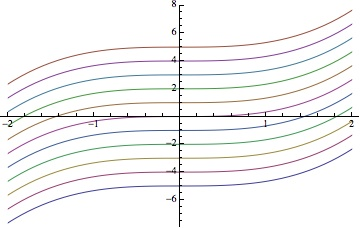# When is an integral not an integral?

Posted by: Gary Ernest Davis on: September 14, 2013No surprise to anyone really that students get confused by the difference between definite and indefinite integrals. The so-called indefinite integral is not really an integral at all, not in the sense of area: it’s the solution set to a differential equation. It’s not even usually a single function at all, but a whole family of functions.

To imagine that$\int x^2 dx$ defines a single thing – a function$g(x)$ for whichÂ$\frac{dg(x)}{dx}=x^2$ Â – is to miss the important point that this differential equation has a whole family of solutions:$\frac{1}{3}x^3+c$, a Â solution for each real number$c \in \mathbb{R}$:So,Â$\int x^2 dx = \{\frac{1}{3}x^3+c \vert c \in \mathbb{R}\}$, NOTÂ$\int x^2 dx =\frac{1}{3}x^3+c$ as is commonly written.

The villain who engendered this confusion was none other than the greatÂ Gottfried Wilhelm Leibniz a co-discover/inventor of calculus along with Isaac Newton.

Wait! I hear you say, isn’t this just pedantry? Does it really matter if we writeÂ$\int x^2 dx$ as$\frac{1}{3}x^3+c$ or as the fashionable set-theoreticÂ$\{\frac{1}{3}x^3+c \vert c \in \mathbb{R}\}$ ?

Well yes, it does. Think about how you might resolve the following apparent conundrum:$\int\frac{1}{x}dx=\int 1\times\frac{1}{x}dx=x\frac{1}{x}+\int x\times\frac{1}{x^2}dx=1+\int \frac{1}{x} dx$ so$0=1$ !!

This is nonsense, of course, because the algebraic calculation proceeds as ifÂ$\int\frac{1}{x}dx$ is a well-defined function, which it is not: it is a family of functions (even worse – defined over a disconnected domain).

Students of mathematics need to learn how to think, not carry out mindless calculations as if they were performing monkeys.

This is an important psychological point: students are used to writingÂ$\int x^2dx=\frac{1}{3}x^3+c$ as a conditioned response, functioning at a symbolic level equivalent to that almost of a dog or a cat (“sit, Fido”, “Here’s food, puss.”), whereas to gain mathematical power and flexibly they need to be functioning at a much higher symbolic level, utilizing a far richer collection of symbolic reference.

### 3 Responses to "When is an integral not an integral?"Interesting. I hadn’t thought about writing it that way. I often work problems to show the students, where the new c in a step is some multiple of the old c, and I carefully label them c sub 1 and c sub 2, and then say I want to be sloppy, is that ok with them. Showing them this set notation might help them see why I do that. You wouldn’t use it all the time, would you?

My bigger issue with the notation is that textbooks introduce indefinite integrals first, and then when they use almost the same notation for area under a curve, students assume anti-derivatives will be involved. So they aren’t suitably impressed with the ideas in the fundamental theorem of calculus. They think it’s obvious, even though they don’t understand it. (The power of notation!)

I have gotten around this by introducing the area using very non-standard notation. I avoid the standard symbols until we’ve gotten through the fundamental theorem.If we agree that C denotes the additive group of constant functions, then$\frac{1}{3}x^3+C$ is a coset. This is similar to how we formalize big-O notation. In your example,$0+C=1+C$ would not be a contradiction.Agreed! And if only I could get sophomore engineering students to think like this!